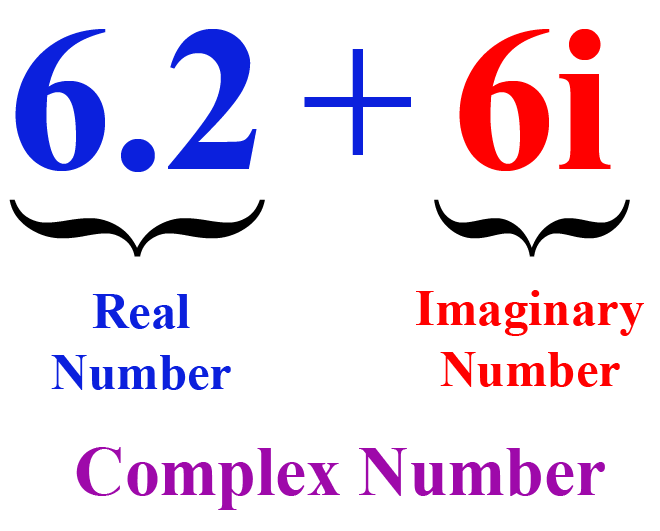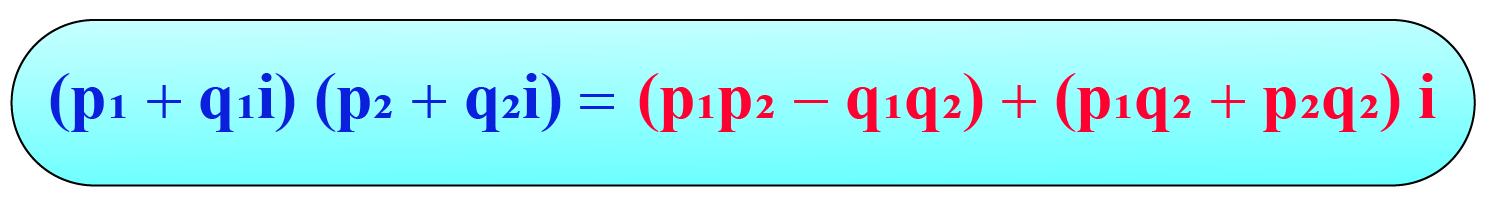# Multiplying Complex Numbers

Multiplying Complex Numbers
Go back to  'Numbers'

Have you ever wondered the outcome of the product of a complex number and imaginary number?

To answer this question, let us understand the basics of real numbers, complex numbers and imaginary numbers.

Natural numbers, rational numbers, decimal numbers and irrational numbers are termed as real numbers.

For example, $$0,1, \dfrac{3}{4}, \pi, 0.235, etc$$.

Imaginary numbers are represented by $$\iota$$. The value of $$i\times i=-1$$ or $$\sqrt{-1}=i$$.

A complex number is a combination of real number and an imaginary number.

For example, $$6.2 + 6i$$In this mini lesson, we will explore the world of multiplication with complex numbers. We will walk through the answers to the questions like how to multiply two complex numbers, how to multiply real number by a complex numbers, how to multiply pure imaginary number by a complex numbers, and how to square a complex number along with solved examples and interactive questions.

## Lesson Plan

 1 How To Multiply Two Complex Numbers? 2 Important Notes on Multiplying Complex Numbers 3 Solved Examples on Multiplying Complex Numbers 4 Interactive Questions on Multiplying Complex Numbers 5 Challenging Question on Multiplying Complex Numbers

## How To Multiply Two Complex Numbers?

Multiplying complex numbers is similar to multiplying polynomials. We use following polynomial identitiy to solve the multiplication.

$$(a+b)(c+d) = ac + ad + bc + bd$$

For multiplying complex numbers we will use the same polynomial identitiy in the follwoing manner.For example, consider two complex numbers (4 + 2i) and (1 + 6i). If you multiply them, then you get:

\begin{align} &=(4 + 2i)(1 + 6i)\\ &=4\times1 + 4\times6i + 2i\times1 + 2i\times6i\\\ &= 4 + 24i + 2i + 12i^2 (\rightarrow i^2 = -1)\\\ &=4+26i-12\\\ &=-8+26i \end{align}

Here, -8 is real part, 26i is an imaginary part of a complex number.

## How To Multiply a Real Number With a Complex Number?

There is a simple way to multiply a real number with a complex number. Follow the example below:

\begin{align}&=2(12+6i)\\ &= 2(12)+(2)(6i)\\ &=24 + 12i \end{align}

Here, 24 is real part, 12i is an imaginary part of a complex number. In this case by using the distributive property we learn how to multiply a real number by a complex number.

Let us leran something more tricky and a bit complicated.

## How To Multiply a Pure Imaginary Number By a Complex Number?

Let us consider an example to learn howto multipy pure imaginary number by a complex number.

For example, multiply $$3i(3-7i)$$ and write the resultig number in a complex form i.e. $$a+bi$$

\begin{align} &=3i(3) - 3i(7i)\\ &=9i + 21i^2\end{align}

We have $$9i + 21i^2$$ as our resultant number. But we need to simplify it more to get the resultant number in the form of $$a+bi$$

We know that,

$$i^{2} = -1$$

\begin{align} &=9i - 21i^{2}\\ &=9i - 21(-1)\\ &=9i + 21\end{align}

With the help of commutative property we can write the resultant number as $$21+9i$$

Hence,

$$3i(3-7i)=21+9i$$

## How To Square a Complex Number?

Squaring a complex number is one of the way to multiply a complex number by itself.

\begin{align} &=(1 + 4i)(1 + 4i)\\ &= 1 + 16i + 16i^{2}\\ &= −15 + 16i\end{align}

Hence,

$$(1+4i)^{2}=-15+16i$$

More Important Topics
Numbers
Algebra
Geometry
Measurement
Money
Data
Trigonometry
Calculus